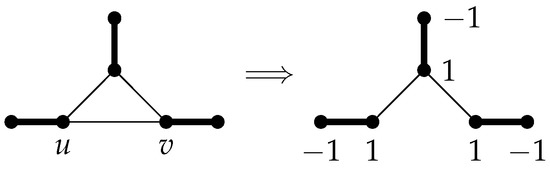Open AccessArticle

# Some Bicyclic Graphs Having 2 as Their Laplacian Eigenvalues

byMasoumeh Farkhondeh 1,Mohammad Habibi 1,Doost Ali Mojdeh 2andYongsheng Rao 3,*1
Department of Mathematics, Tafresh University, Tafresh 39518-79611, Iran
2
Department of Mathematics, University of Mazandaran, Babolsar 47416-95447, Iran
3
Institute of Computing Science and Technology, Guangzhou University, Guangzhou 510006, China
*
Author to whom correspondence should be addressed.
Mathematics 2019, 7(12), 1233; https://doi.org/10.3390/math7121233
Received: 13 November 2019 / Revised: 8 December 2019 / Accepted: 9 December 2019 / Published: 12 December 2019
If G is a graph, its Laplacian is the difference between the diagonal matrix of its vertex degrees and its adjacency matrix. A one-edge connection of two graphs $G 1$ and $G 2$ is a graph $G = G 1 ⊙ u v G 2$ with $V ( G ) = V ( G 1 ) ∪ V ( G 2 )$ and $E ( G ) = E ( G 1 ) ∪ E ( G 2 ) ∪ { e = u v }$ where $u ∈ V ( G 1 )$ and $v ∈ V ( G 2 )$ . In this paper, we study some structural conditions ensuring the presence of 2 in the Laplacian spectrum of bicyclic graphs of type $G 1 ⊙ u v G 2$ . We also provide a condition under which a bicyclic graph with a perfect matching has a Laplacian eigenvalue 2. Moreover, we characterize the broken sun graphs and the one-edge connection of two broken sun graphs by their Laplacian eigenvalue 2. View Full-Text
Keywords:
Show FiguresFigure 1

MDPI and ACS Style

Farkhondeh, M.; Habibi, M.; Mojdeh, D.A.; Rao, Y. Some Bicyclic Graphs Having 2 as Their Laplacian Eigenvalues. Mathematics 2019, 7, 1233.